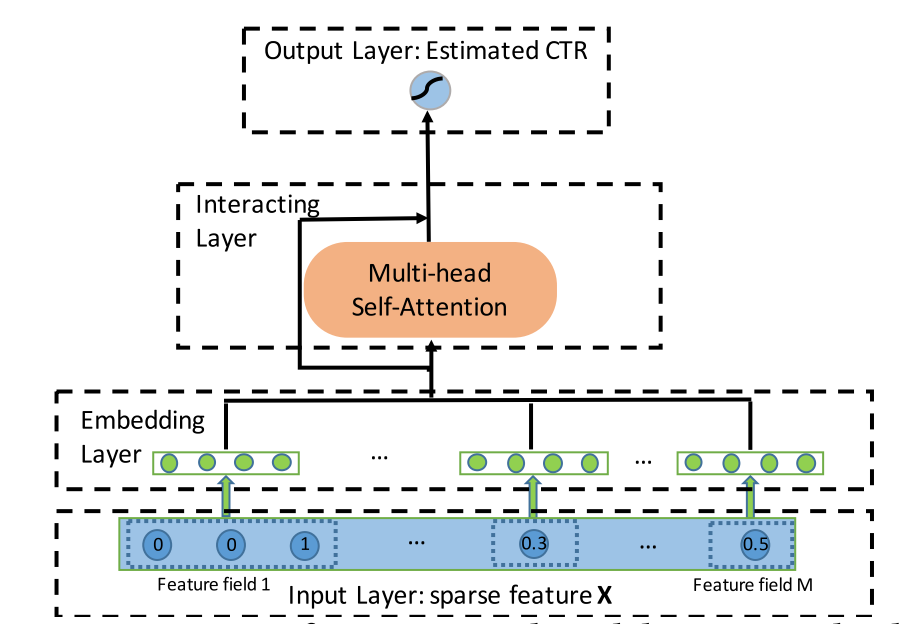## 输入和嵌入

1. 进行归一化处理拼接到embedding向量侧
2. 进行离散化处理作为类别特征
3. 赋予其一个embedding向量，每次用特征值与embedding向量的乘积作为其最终表示

## InteractingLayer（交互层）

1. 首先输入特征通过矩阵乘法线性变换为在注意力空间下的向量表示，对于每个特征在特定的注意力空间中，都有三个表示向量,,2. 计算与其他特征的相似度，本文使用向量内积表示：3. 计算softmax归一化注意力分布：4. 通过加权求和的方式得到特征及其相关的特征组成的一个新特征## 我不想看数学，我想看代码：OK

1. 通过矩阵乘法得到注意力空间下的三组向量表示
querys = tf.tensordot(inputs, W_Query, axes=(-1, 0))  # (batch_size,field_size,att_embedding_size*head_num)
keys = tf.tensordot(inputs, W_key, axes=(-1, 0))
values = tf.tensordot(inputs, W_Value, axes=(-1, 0))

2. 为了同时在不同的子空间下计算特征相似度，需要先进行一些变换

querys = tf.stack(tf.split(querys, self.head_num, axis=2))  # (head_num,batch_size,field_size,att_embedding_size)
values = tf.stack(tf.split(values, self.head_num, axis=2))

3. 计算相似度及归一化注意力分布

inner_product = tf.matmul(querys, keys, transpose_b=True)  # (head_num,batch_size,field_size,field_size)
normalized_att_scores = tf.nn.softmax(inner_product)

4. 计算加权和

result = tf.matmul(normalized_att_scores, values)  # (head_num,batch_size,field_size,att_embedding_size)

5. 将不同子空间下的结果进行拼接

result = tf.concat(tf.split(result, self.head_num, ), axis=-1)
result = tf.squeeze(result, axis=0)# (batch_size,field_size,att_embedding_size*head_num)

6. 使用残差连接保留原始信息，

if use_res:
result += tf.tensordot(inputs, self.w_res, axes=(-1, 0))
result = tf.nn.relu(result)# (batch_size,field_size,att_embedding_size*head_num)

## 我不想看代码，我想直接拿来用：没问题

import pandas as pd
from sklearn.metrics import log_loss, roc_auc_score
from sklearn.model_selection import train_test_split
from sklearn.preprocessing import LabelEncoder, MinMaxScaler

from deepctr.models import AutoInt
from deepctr.inputs import  SparseFeat, DenseFeat, get_feature_names

if __name__ == "__main__":

sparse_features = ['C' + str(i) for i in range(1, 27)]
dense_features = ['I' + str(i) for i in range(1, 14)]

data[sparse_features] = data[sparse_features].fillna('-1', )
data[dense_features] = data[dense_features].fillna(0, )
target = ['label']

# 1.Label Encoding for sparse features,and do simple Transformation for dense features
for feat in sparse_features:
lbe = LabelEncoder()
data[feat] = lbe.fit_transform(data[feat])
mms = MinMaxScaler(feature_range=(0, 1))
data[dense_features] = mms.fit_transform(data[dense_features])

# 2.count #unique features for each sparse field,and record dense feature field name

fixlen_feature_columns = [SparseFeat(feat, data[feat].nunique())
for feat in sparse_features] + [DenseFeat(feat, 1,)
for feat in dense_features]

dnn_feature_columns = fixlen_feature_columns
linear_feature_columns = fixlen_feature_columns

feature_names = get_feature_names(linear_feature_columns + dnn_feature_columns)

# 3.generate input data for model

train, test = train_test_split(data, test_size=0.2)
train_model_input = {name:train[name] for name in feature_names}
test_model_input = {name:test[name] for name in feature_names}

# 4.Define Model,train,predict and evaluate
metrics=['binary_crossentropy'], )

history = model.fit(train_model_input, train[target].values,
batch_size=256, epochs=10, verbose=2, validation_split=0.2, )
pred_ans = model.predict(test_model_input, batch_size=256)
print("test LogLoss", round(log_loss(test[target].values, pred_ans), 4))
print("test AUC", round(roc_auc_score(test[target].values, pred_ans), 4))



（本文贴图取自于该论文）